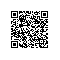# noip2014普及组 比例简化

1498 902 10

5 31 #include<stdio.h>
2 int gcd(int a,int b);
3 int main()
4 {
5     int a,b,L;
6     int i,j;
7     int mina,minb;
8     double  min,tt,t;
9     //freopen("ratio.in","r",stdin);
10     //freopen("ratio.ans","w",stdout);
11     scanf("%d%d%d",&a,&b,&L);
12     t=a*1.0/b;
13     min=200;
14     for(i=1;i<=L;i++)
15     {
16         for(j=1;j<=L;j++)
17         {
18             tt=i*1.0/j;
19             if(tt>=t&&tt<min&&gcd(i,j)==1)
20             {
21                 min=tt;
22                 mina=i;
23                 minb=j;
24             }
25         }
26     }
27     printf("%d %d\n",mina,minb);
28     return 0;
29 }
30 int gcd(int a,int b)
31 {
32     int t;
33     if(a<b)
34     {
35         t=a;a=b;b=t;
36     }
37     /*if(b==0) return a;
38     else return gcd(b,a%b);*/
39     t=a%b;
40     while(t!=0)
41     {
42         a=b;
43         b=t;
44         t=a%b;
45     }
46     return b;
47 }
View Code使用钉钉扫一扫加入圈子
+ 订阅